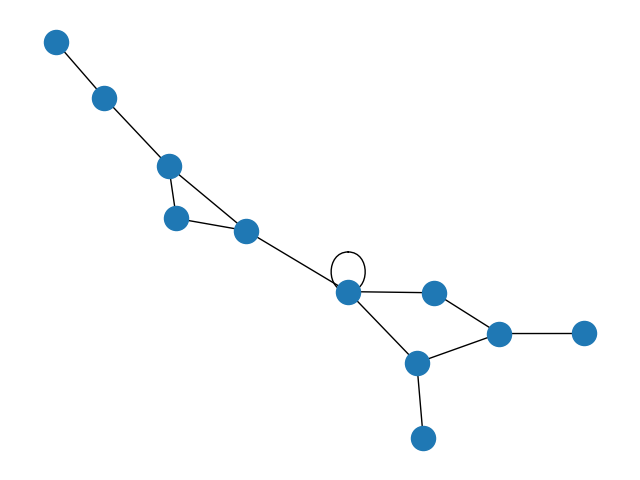# Degree Sequence¶

Random graph from given degree sequence.Out:

```True
Configuration model
Degree sequence [5, 3, 3, 3, 3, 2, 2, 2, 1, 1, 1]
Degree histogram
degree #nodes
5      1
3      4
2      3
1      3
```

```import matplotlib.pyplot as plt
import networkx as nx

# Specify seed for reproducibility
seed = 668273

z = [5, 3, 3, 3, 3, 2, 2, 2, 1, 1, 1]
print(nx.is_graphical(z))

print("Configuration model")
G = nx.configuration_model(z, seed=seed)  # configuration model, seed for reproduciblity
degree_sequence = [d for n, d in G.degree()]  # degree sequence
print(f"Degree sequence {degree_sequence}")
print("Degree histogram")
hist = {}
for d in degree_sequence:
if d in hist:
hist[d] += 1
else:
hist[d] = 1
print("degree #nodes")
for d in hist:
print(f"{d:4} {hist[d]:6}")

pos = nx.spring_layout(G, seed=seed)  # Seed layout for reproducibility
nx.draw(G, pos=pos)
plt.show()
```

Total running time of the script: ( 0 minutes 0.072 seconds)

Gallery generated by Sphinx-Gallery